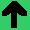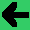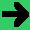# Lab 10: Driving With Acceleration

1. Lets change the game so that it has acceleration.
2. Add a velocity vector. Initially this is zero.
3. Add an acceleration vector also initially zero.
4. Make velocity change position. (4 points)
5. Change the up and down from movement to acceleration.(1 point)
6. Make acceleration change velocity. (1 point)
7. Make it stop when it runs off the track.(2 points)
8. Try to integrate traction. First try constant deceleration, (1 point)
9. then try friction (I got it working with constant deceleration). (1 point)